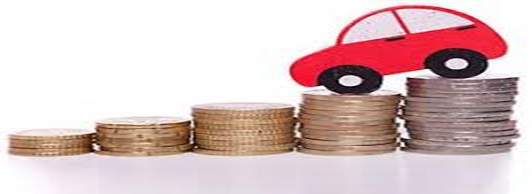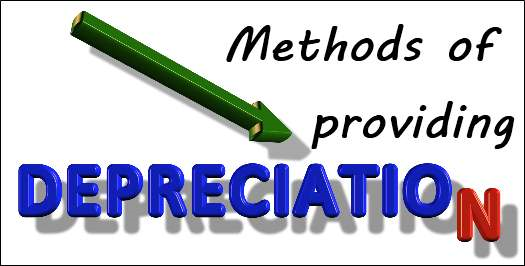DepreciationDepreciation is a particular method wherein, there is a gradual reduction in the value of fixed assets as a result of regular wear and tear in the course of the value of assets. This is a method of allocation of the cost of capital of assets throughout the useful life of the asset. Depreciation is an expense or cost which is being associated with the revenue which is being generated during the reporting year. The effect is being reflected in the declined value of the asset. The accounting entry for finding out depreciation is as follows. Depreciation expenses a/c Dr. Accumulated depreciation ac cr a. Accelerated depreciation: Most of the expenses are being allocated in the first few years of putting the asset to use. The productivity of certain assets is being used for the purpose of ensuring that, tasks are being taken into consideration.

Depreciation expenses a/c Dr

Accumulated depreciation ac cr

a Accelerated depreciation: Most of the expenses are being allocated in the first few years of putting the asset to use. The productivity of certain assets is being used for the purpose of ensuring that, tasks are being taken into consideration.

b. Group assets: These are the assets which are similar in function to each other and are being pooled together as these assets have a similar life.

c. Composite assets: These are the assets which are not similar to each other but the functions are being such which can be clubbed in a specific category.

Methods of Depreciation· Straight line method: In this method, each of the accounting years is being allocated the specific value of depreciation. The value of depreciation is being deducted each year and equal value is being deducted on regular basis.

· Written down value method: This is a method which is also being called as reducing balance method. In this method, the depreciation is being calculated at a particular rate but the value of the asset is being reduced every year and depreciation is being charged on the reduced value every year.

· Units of production depreciation: This method is also being termed as units or usage or units of activity. It makes a measurement of the currently achieved level of output to the expected level of output. In this method, the useful life of the asset is being calculated in terms of output of production and not on the basis of years of service. The total estimated output or usage of an asset is to be taken into consideration during the usage of the product is taken into account.

Example: A factory installed a packaging plant costing \$ 20 million and it is expected capacity is 80 million packages over its period of life. Its residual value is \$2 million. The packaging in the first year is expected to be 10 million packages. Thus the depreciation for the first year is calculated as below:

Sum of the year’s digit method: This type of depreciation method is being similar in comparison to reducing to balance method. It is being charged on the fractional element of the total sum of the years.

Example: If the life of the asset is 3 years, then the sum of the assets is 1 + 2 + 3 = 6.

The fractional part is the year under consideration to calculate depreciation and it is the non-depreciated useful life of the asset.

For year 1= depreciation is 3/6 * depreciable cost

For year 2= depreciation is 2/6 * depreciable cost

For year 3= depreciation is 1/6 * depreciable cost

Reasons for Depreciation

·         There is the occurrence of wear and tear in the asset as a result of its regular usage.

·         It is being identified that whether the asset is there in use or not, there is always a case of deterioration in the value of the asset

·         There are certain assets which come with usage rights, therefore, it is essential to provide depreciation for such assets.

·         There can be incidences such as fire, natural calamities, and accidents etc.

·         Mishandling of assets

·         The technology is dynamic and ever-changing

·         Depletion can arise in the asset in the course of usage of the asset to a larger level.

Depreciation is a particular method wherein, there is a gradual reduction in the value of fixed assets as a result of regular wear and tear in the course of the value of assets. This is a method of allocation of the cost of capital of assets throughout the useful life of the asset. Depreciation is an expense or cost which is being associated with the revenue which is being generated during the reporting year. The effect is being reflected in the declined value of the asset. The accounting entry for finding out depreciation is as follows.

Depreciation expenses a/c Dr

Accumulated depreciation ac cr

a.       Accelerated depreciation: Most of the expenses are being allocated in the first few years of putting the asset to use. The productivity of certain assets is being used for the purpose of ensuring that, tasks are being taken into consideration.

b.      Group assets: These are the assets which are similar in function to each other and are being pooled together as these assets have the similar life.

c.       Composite assets: These are the assets which are not similar to each other but the functions are being such which can be clubbed in a specific category.

If you need more help in depreciation-methods

## Phoenix## Genius## Vikas